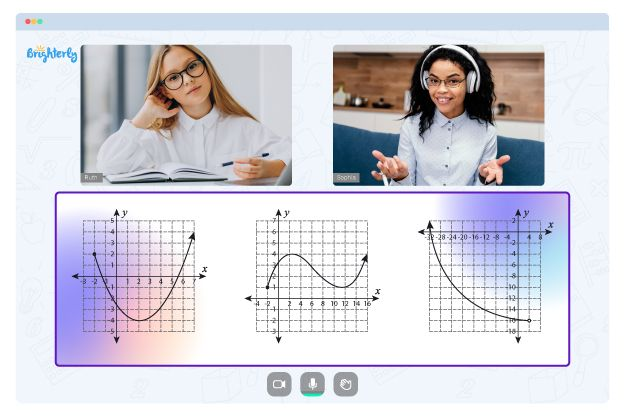# Coordinate Plane Worksheets

You can plot points, graph equations, and solve problems in two-dimensional space applying the knowledge of the coordinate plane mathematical concept. Moreover, numerous fields, such as engineering, physics, and computer science, use the coordinate plane. But what about teaching it?

Teachers frequently use coordinate plane worksheets to assist students in mastering the skills needed to work with the coordinate plane. These worksheets reinforce the knowledge needed to solve questions around the concept.

## Worksheets on Coordinate Planes

The coordinate plane is a two-dimensional space that lets us represent and evaluate geometrical figures and mathematical relationships. The origin is the point at which the x-axis and y-axis meet, and its coordinates are (0, 0). Negative values extend to the left of the origin, while positive values extend to the right of the origin. From the origin, positive values on the y-axis extend upward, whereas negative values extend downward.

Using coordinate plane graphing worksheets, students can practice their numerical skills, like tracking down the distance between two focuses, deciding the midpoint of a line fragment, and recognizing the incline of a line. In addition, they can investigate mathematical ideas like evenness, interpretations, revolutions, and reflections.

These worksheets are meant to help students improve their algebra and geometry skills, as well as their spatial reasoning and problem-solving abilities. In addition, students can increase their confidence in math knowledge and comprehension of mathematical concepts by engaging with the coordinate plane in a visual and hands-on manner.

## How to Study with Worksheets on the Coordinate Plane

One way to use coordinate plane worksheets is to show students how to distinguish and chart functions. They can use coordinate plane worksheets PDF to improve their spatial reasoning skills. For example, students can work on their capacity to envision and intellectually manipulate objects in a three-dimensional space. Success in physics, engineering, and architecture depends on this ability.

Math for Kids

Is Your Child Struggling With Math?
1:1 Online Math TutoringFree coordinate plane worksheets with larger grid sizes and more explicit labeling may benefit students who struggle with visual-spatial reasoning. Likewise, students who succeed in arithmetic might benefit from more complicated worksheets that challenge them to apply their insight in new and imaginative ways.

Worksheets on coordinate planes can be fun. They reinforce what students have learned in the classroom. They contain coordinate plane puzzles or challenges that require students to use their critical thinking and problem-solving abilities. When assigned by teachers, those puzzles keep students motivated and interested in learning.

## Advantages of Utilizing Coordinate Plane Worksheets

The coordinate plane worksheets 5th grade students use are excellent promoters of cooperative learning environments. By working together while solving worksheets’ problems, students can share their knowledge and understanding of mathematical concepts, learn from each other’s experiences, and build community and support. Collaborative learning also helps students develop essential skills for working in teams, such as communication and problem-solving.

For visual learners, coordinate plane worksheets picture are handy tools. They allow learners to perceive mathematical concepts visually and tangibly, which can help them better understand abstract ideas and make connections between mathematical concepts. In addition, using graphs and charts allows visual learners to deepen their understanding of mathematical concepts and improve their ability to visualize mathematical relationships.

The use of coordinate plane picture worksheets also allows students to engage in hands-on learning. By physically manipulating points and lines on the coordinate plane, kids can deepen their understanding of mathematical concepts and develop a sense of ownership and control over their learning. Thus, they stay engaged and motivated in their learning.

The skills students acquire through working with coordinate plane worksheets PDF and answers are highly adaptable and can be applied to various academic fields and real-world scenarios.

Need help with Coordinate Plane?• Is your child finding it challenging to understand the concept of coordinate plane?
• An online tutor could be the answer.

Does your child struggle to grasp coordinate plane lessons? Try studying with an online tutor.